# SPSS tutorials

BASICS DATA ANALYSIS T-TEST ANOVA CHI-SQUARE TEST

# SPSS Binomial Test Tutorial

Also see Binomial Test - Simple Tutorial for a quick explanation of how this test works.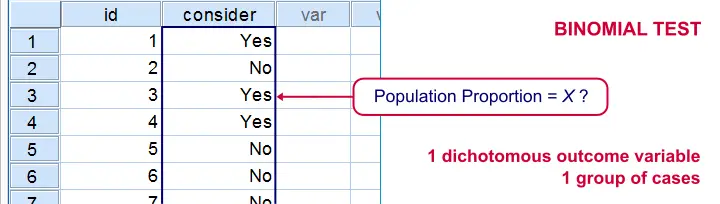SPSS binomial test is used for testing whether a proportion from a single dichotomous variable is equal to a presumed population value. The figure illustrates the basic idea.

## SPSS Binomial Test Example

A biologist claims that 75% of a population of spiders consist of female spiders. With a lot of effort he collects 15 spiders, 7 of which are female. These data are in spiders.sav, part of which are shown below.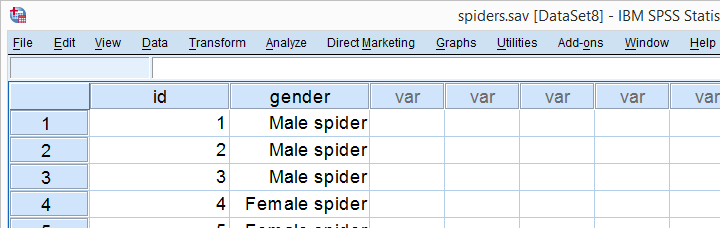## 1. Quick Data Check

Let's first take a quick look at the FREQUENCIES for gender. Like so, we can inspect whether there are any missing values and whether the variable is really dichotomous. We'll run some FREQUENCIES. The syntax is so simple that we'll just type it instead of clicking through the menu.

*Inspect frequency distribution.

frequencies gender.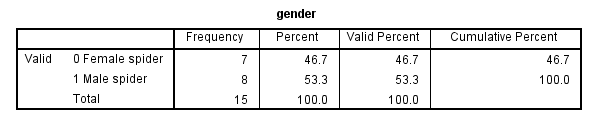The output tells us that there are no missing values and the variable is indeed dichotomous. We can proceed our analysis with confidence.

## 2. Assumptions Binomial Test

The results from any statistical test can only be taken seriously insofar as its assumptions have been met. For the binomial test we need just one:

• independent observations (or, more precisely, independent and identically distributed variables);

This assumption is beyond the scope of this tutorial. We presume it's been met by the data at hand.

## 3. Run SPSS Binomial Test

We'd like to test whether the proportion of female spiders differs from .75 (our test proportion). Now SPSS Binomial Test has a very odd feature: the test proportion we enter applies to the category that's first encountered in the data. So the hypothesis that's tested depends on the order of the cases. Because our test proportion applies to female (rather than male) spiders, we need to move our female spiders to the top of the data file. We'll do so by running the syntax below. Next, we'll run the actual binomial test.

*Move Female Spiders to Top of File.

sort cases by gender.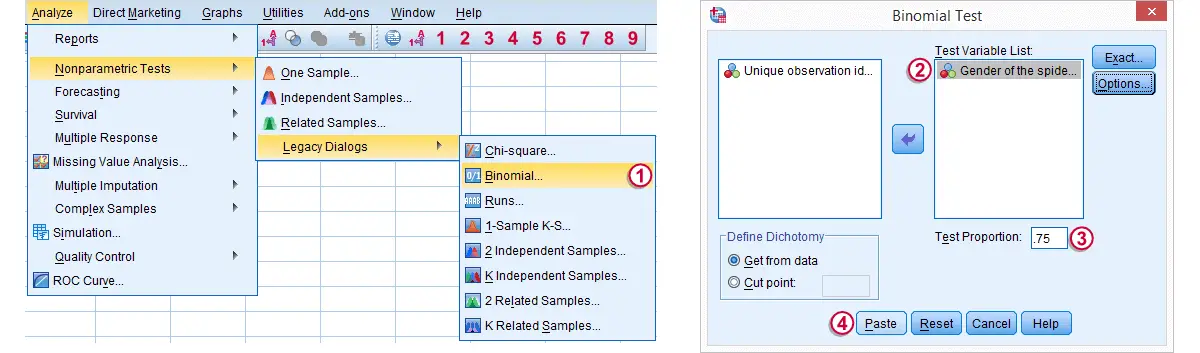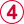Clicking results in the syntax below. We'll run it and move on the the output.

*Run SPSS Binomial Test.

NPAR TESTS
/BINOMIAL (.75)=gender
/MISSING ANALYSIS.

## 4. SPSS Binomial Test Output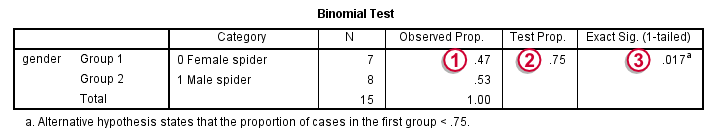Since we have 7 female spiders out of 15 observations, the observed proportion is (7 / 15 =) .47.Our null hypothesis states that this proportion is .75 for the entire population.The p value, denoted by Exact Sig. (1-tailed) is .017. If the proportion of female spiders is exactly .75 in the entire population, then there's only a 1.7% chance of finding 7 or fewer female spiders in a sample of N = 15. We often reject the null hypothesis if this chance is smaller than 5% (p < .05). We conclude that the proportion of female spiders is not .75 in the population but probably (much) lower.
Note that the p value is the chance of finding the observed proportion or a “more extreme” outcome. If the observed proportion is smaller than the test proportion, then a more extreme outcome is an even smaller proportion than the one we observe.The reasoning is entirely reversed when the observed proportion is larger than the expected proportion. We ignore the fact that finding very large proportions would also contradict our null hypothesis. This is what's meant by (1-tailed).A 2-tailed binomial test is only be applied when the test proportion is exactly .5. The (rather technical) reason for this is that the binomial sampling distribution for the observed proportion is only symmetrical in the latter case.

## 5. Reporting a Binomial Test

When reporting test results, we always report some descriptive statistics as well. In this case, a frequency table will do. Regarding the significance test, we'll write something like “a binomial test indicated that the proportion of female spiders of .47 was lower than the expected .75, p = .017 (1-sided)”.

# Let me know what you think!

*Required field. Your comment will show up after approval from a moderator.

# This tutorial has 34 comments

•### By Ruben Geert van den Berg on July 16th, 2016

Hi Hoang!

This is because you skipped at least 1 step explained in this tutorial: you didn't run SORT CASES. If you don't, then you're testing a different hypothesis (75% male instead of female spiders) than the one mentioned in this tutorial and hence you get a different p-value. The 0.057 is the probability of finding 8 or fewer male spiders under the (wrong) null hypothesis that 75% of our spiders are male.

•### By Hoang Dinh Hue on July 15th, 2016

Dear You,
I had applied the "Run SPSS Binomial Test" as you showed but I have received the different results:
The Exact Sig. (1-sign) is 0.057 , it is different to your result 0.017?
Please explain it to me
Thank you a lot
Hoang dinh Hue

•### By Ruben Geert van den Berg on April 19th, 2016

Hi Ing!

It's a really good question so I may actually write a tutorial on it. Until then, try the example at SPSS - Confidence Interval Single Proportion from T-Test.

I threw in a lot of comments regarding the outcomes and SPSS menu navigation. Hope that helps!

•### By Ing on April 19th, 2016

Thank you for the promt reply.
My N varies between 40 and 87, so this would be fine, but I am a little bit confused how I can use a t-test for a dichotomous variable (YES/NO). But I thought that there should be a confidence interval for proportion of a dichotomous variable as well... Would be great if you can help again :)

•### By Ruben Geert van den Berg on April 19th, 2016

Hi Ing!

If your sample size is large enough (rules of thumb vary from n > 30 to n > 50), then you can use a one sample t-test instead of a binomial test. The one-sample t-test will provide you with a confidence interval for a proportion.

Strictly, you should use a z-test instead but for reasonable sample sizes, its results are basically identical to the aforementioned t-test as well as the binomial test (same outcome for all three, which makes perfect sense). I believe the z-test for proportions is not present in SPSS for precisely this reason.